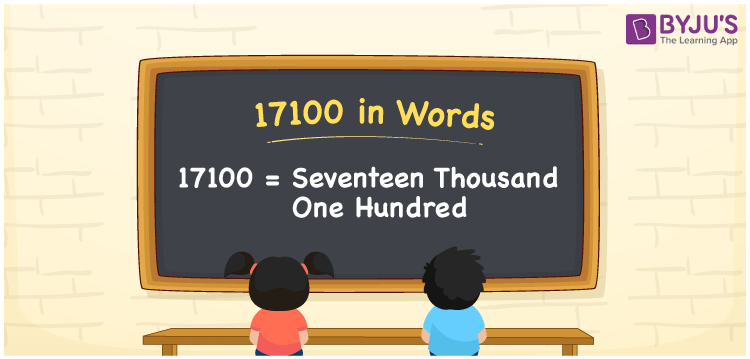# 17100 in Words

17100 in words can be written as Seventeen Thousand One Hundred. The fundamental concepts in Mathematics like counting or count can be learnt efficiently here. If you buy a dining table for Rs. 17100, then you can say that “I bought a dining table for Seventeen Thousand One Hundred Rupees”. To write numbers in words, the English alphabet is used. The numbers in words concept is explained here in a simple way to improve the conceptual knowledge of students. The 17100 can be read as “Seventeen Thousand One Hundred” in English.

 17100 in words Seventeen Thousand One Hundred Seventeen Thousand One Hundred in Numbers 17100

## 17100 in English Words## How to Write 17100 in Words?

Students can learn about the expanded form and the place value chart of 17100. Five digits are present in the number 17100. With the help of the place value chart given below, students will be able to understand the concepts with ease.

 Ten Thousands Thousands Hundreds Tens Ones 1 7 1 0 0

17100 can be written in expanded form as:

1 x Ten Thousand + 7 x Thousand + 1 × Hundred + 0 × Ten + 0 × One

= 1 x 10000 + 7 x 1000 + 1 × 100 + 0 × 10 + 0 × 1

= 10000 + 7000 + 100

= 17100

= Seventeen Thousand One Hundred

Hence, 17100 in words is written as Seventeen Thousand One Hundred.

17100 is a natural number that precedes 17101 and succeeds 17099.

17100 in words – Seventeen Thousand One Hundred

Is 17100 an odd number? – No

Is 17100 an even number? – Yes

Is 17100 a perfect square number? – No

Is 17100 a perfect cube number? – No

Is 17100 a prime number? – No

Is 17100 a composite number? – Yes

## Frequently Asked Questions on 17100 in Words

Q1

### How to write 17100 in words?

17100 can be written in words as “Seventeen Thousand One Hundred”.
Q2

### How to write Seventeen Thousand One Hundred in numbers?

Seventeen Thousand One Hundred in numbers can be written as 17100.
Q3

### Is 17100 an odd or even number?

17100 is an even number as it is completely divisible by 2.
17100/2 = 8550